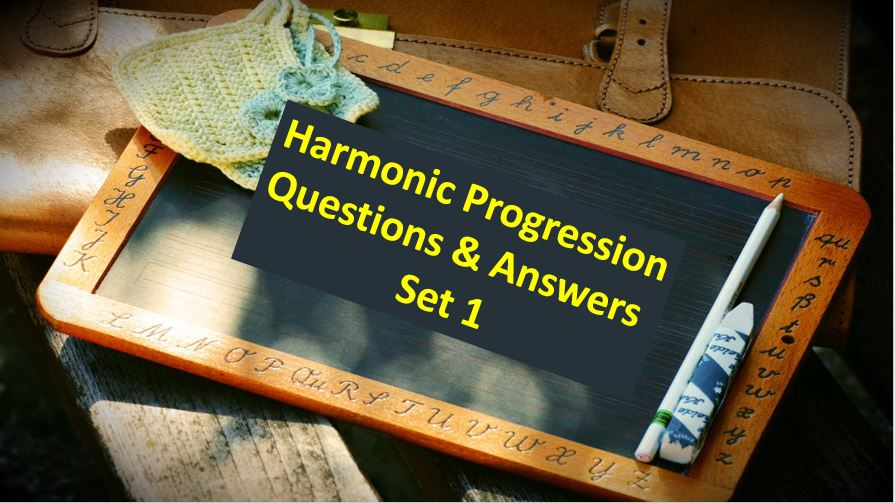Monday, September 27, 2021
Home > Question and Answer > Harmonic Progression Question And Answer Set 1

# Harmonic Progression Question And Answer Set 1# Harmonic Progression Question and Answer Set 1

Hi students, welcome to Amans Maths Blogs (AMB). On this post, you will get the Harmonic Progression Question and Answer Set 1 is the collection of some important questions. Practice these questions for SSC CGL CHSL CAT NTSE exams etc. It will help you to practice the questions on the topics of maths as harmonic progression based questions of algebra.

Harmonic Progression Question and Answer Set 1: Ques No 1

In HP, the sum of first two terms is 17/70, the sum of next two terms is 5/4, the sum of following next two terms is -7/10. The sum of following next two terms is

Options:

A. -64/75

B. -18/63

C. -19/88

D. None of these

Harmonic Progression Question and Answer Set 1: Ques No 2

In HP, the 2nd term of an HP is 40/9 and the 5th term is 20/3. Find the maximum possible number of terms in H.P.

Options:

A. 9

B. 10

C. 11

D. 12

Harmonic Progression Question and Answer Set 1: Ques No 3

If a, b, c are in HP, then a/(b + c), b/(c + a), c/(a + b) are in

Options:

A. AP

B. GP

C. HP

D. None of these

Harmonic Progression Question and Answer Set 1: Ques No 4

A person drives a car from A to B with a speed of 60 km/hr and returns from B to A with a speed of 40 km/hr. What is the average speed for the whole journey?

Options:

A. 40 km/h

B. 50 km/h

C. 48 km/h

D. 52 km/h

Harmonic Progression Question and Answer Set 1: Ques No 5

The sum of the reciprocals of three numbers in HP is 12 and the product of the number is 1/48. The second number is

Options:

A. 1/2

B. 1/4

C. 1/6

D. None of these

Harmonic Progression Question and Answer Set 1: Ques No 6

If a1, a2, a3, … an are in HP, then the sum of a1a2 + a2a3 + … + an-1an is

Options:

A. a1an

B. na1an

C. (n – 1)a1an

D. None of these